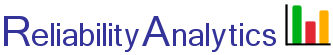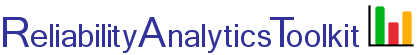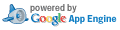# Normal Distribution

The normal distribution can be used to model the reliability of items that experience wearout failures. Given a mean life, μ and standard deviation, σ, the reliability can be determined at a specific point in time (t). The normal distribution probability density function, reliability function and hazard rate are given by: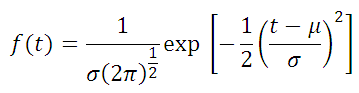Probability Density Function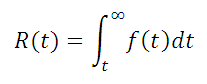Reliability Function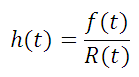Hazard Rate

For example, given a mean life of a light bulb of μ=900 hours, with a standard deviation of σ=300 hours, the reliability at the t=700 hour point is 0.75, as represented by the green shaded area in the picture below.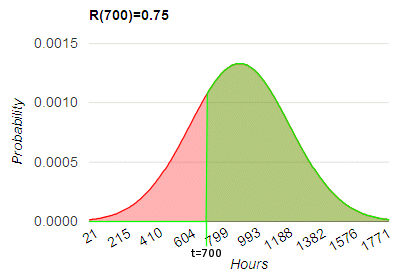## Calculation Inputs:

1. Mean life (μ):
2. Standard deviation (σ):
3. Time period of interest (t):
4. Units associated with inputs #1, #2 and #3 above:
5. Decimal places:
6. Options:

Table
f(t) chart
R(t) chart
F(t) chart
h(t) chart

Title (optional):

## Featured Reference: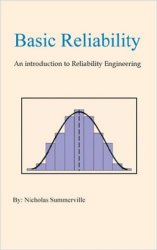Basic Reliability: An introduction to Reliability Engineering

Toolkit Home